Plotting Complex NumbersCustom SearchPlotting Complex Numbers Complex numbers may easily be plotted in the complex plane. Pure imaginaries are plotted along the vertical axis, the axis of imaginaries, and real numbers are plotted along the horizontal axis, the axis of reals. It follows that other points in the complex plane must represent numbers that are part real and part imaginary; in other words, complex numbers. If we wish to plot the point 3 + 2i, we note that the number is made up of the real number 3 and the imaginary number 2i. Thus, as in figure 15-8, we measure along the real axis in a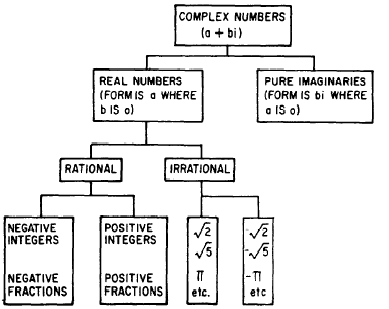Figure 15-7. -The complex number system.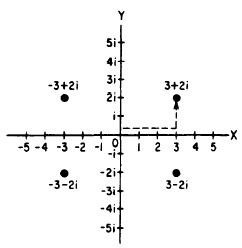Figure 15-8.-Plotting complex numbers.  positive direction. At point (3, 0) on the real axis we turn through one right angle and measure 2 units up and parallel to the imaginary axis. Likewise, the number -3 + 2i is 3 units to the left and up 2 units; the number 3 - 2i is 3 units to the right and down 2 units; and the number -3 -2i is 3 units to the left and down 2 units. Complex Numbers as Vectors A vector is a directed line segment. A complex number represents a vector expressed in the RECTANGULAR FORM. For example, the complex number 6 + 8i in figure 15-9 may be considered as representing either the point P or the line OP. The real parts of the complex number (6 and 8) are the rectangular components of the vector. The real parts are the legs of the right triangle (sides adjacent to the right angle), and the vector OP is its hypotenuse (side opposite the right angle). If we merely wish to indicate the vector OP, we may do so by writing the complex number that represents it along the segment as in figure 15-9. This method not only fixes the position of point P, but also shows what part of the vector is imaginary (PA) and what part is real (OA). If we wish to indicate a number that shows the actual length of the vector OP, it is necessary ’ to solve the right triangle OAP for its hypotenuse. This may be accomplished by taking the square root of the sum of the squares of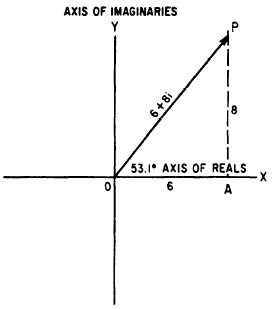Figure 15-9. -A complex number shown as a vector. the legs of the triangle, which in this case are the real numbers, 6 and 8. thus,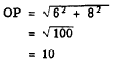However, since a vector has direction as well as magnitude, we must also show the direction of the segment; otherwise the segment OP could radiate in any direction on the complex plane from point 0. The expression 10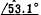. 10 indicates that the vector OP has been rotated counterclockwise from the initial positibn through an angle of 53.1°. (The initial position in a line extending from the origin to the right along OX.) This method of expressing the vector quantity is called the POLAR FORM. The number represents the magnitude of the quantity, and the angle represents the position of the vector with respect to the horizontal reference, OX. Positive angles. represent counterclockwise rotation of the vector, and negative angles represent clockwise rotation. The polar form is generally simpler for multiplication and division, but its use requires a knowledge of trigonometry.Integrated Publishing, Inc. - A (SDVOSB) Service Disabled Veteran Owned Small Business